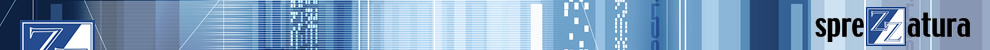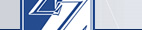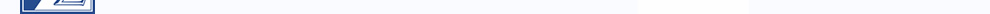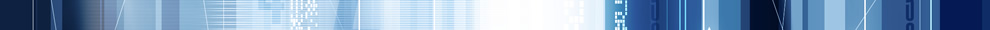By Sprezz | Thursday, 12 March 2020 14:48 | 0 Comments
We've recently been supporting a venerable app for a long time Revelation Client (put it this way, when working on it recently, we came across some of our code taken from a REVMEDIA article nearly 30 years ago!) whose developer has retired.

Year end had come around and some modifications made in the last decade had finally turned around to bite the client. Think of a Y2K bug and then increase its occurrence by two orders of magnitude.

The system - as part of its year end routine - would calculate the starting row id number for a specific temporary table, by taking the last digit of the year and multiplying it by 10,000. It would then drop this into the row id prompt automatically. So for 2017 the starting number would be 7 * 10,000 = 70,000. For 2019, 9 * 10,000 = 90,000. For 2020? Less good. 0 * 10,000 = 0.

Of course, we could get around that issue if it were not for the fact that this calculation was done on the default row id prompt, which also had a validation of 5N.

So the system calculated a starting number of 0, and OI rejected it as not being a 5 digit number. So the system calculated a starting number of 0, and OI rejected it as not being a 5 digit number. So the system calculated a starting number of 0, and OI rejected it as not being a 5 digit number. So the system calculated a starting number of 0, and OI rejected it as not being a 5 digit number. So the system calculated a starting number of 0, and OI rejected it as not being a 5 digit number. So the system calculated a starting number of 0, and OI rejected it as not being a 5 digit number. So the system calculated a starting number of 0, and OI rejected it as not being a 5 digit number. So the system calculated a starting number of 0, and OI rejected it as not being a 5 digit number..... you get the picture.

That was a relatively easy fix once the business decided what they wanted to use instead.

The bug that REALLY threw us, was that in other parts of the system, we would enter a new starting row id, the system would accept it, look up the row and then reset the row id to a seemingly random number. Initially our suspicion was raised by the default program and we took some time analysing that but couldn't see where the issue lay. This being AREV32 we had lots of potential culprits... was it the
•  Post-prompt routine
•  Pre-prompt routine
•  Post-app initialise routine (This did a LOT of work rearranging prompts and prompt logic)
Whatever it was it wasn't screaming out to be identified, but after a couple of hours of debugging we zoomed in on the fact that the pre-prompt routine used a parameter row called 00 to store the defaults.

We opened the parameter row up in the editor and it existed alright - so we updated the defaults in that row, saved them and went off to see if our fix had worked.  We were taken aback to see that it didn't. Despite putting 100,000 into the correct position in the parameter row, the seemingly random number kept turning up like a bad penny.

So we zoomed in on the section of code that grabbed the default number :-

ReadV defaultNum from vTable, 00, 978 else call fsMsg()

We double-checked, and yes, 100,000 was indeed in column 978 of row 00. We triple-checked. No change. And then, because we now knew where the problem lay, it became obvious. One of those "Well d'uh" moments.

Opening up the editor we looked to see if there was a parameter row with a row id of 0. Of course there was. And in CAPITAL LETTERS across the top of of the first column was "DEPRECATED - DO NOT USE".  And there in column 978 of row 0 was our seemingly random number.

With hindsight this was so obvious - the 00 was not quoted so wasn't treated as a string, it was treated as a number, and 00 === 0 if we're talking numbers. But it just seemed so strange that a developer would deliberately choose to use 00 over 0 if they meant 0. Of course, the strong likelihood is that the developer thought that they meant 00 - hence the comment in column 1 of row 0!

We even went back to original AREV on an XP (thanks Carl and Bill) to check the original behaviour, and unsurprisingly nothing has changed. So mystery solved and problem averted but a salutary lesson.

<< Home

Previous Posts
Archives
BlogRollSubscribe to
Posts [Atom]Making Databases Happen UK +44 (0) 20 8912 1010 USA +1 267 238 3814